# Fraction Simplifier – Fraction Reducer

Enter a fraction below to simplify or reduce it to the simplest form. The calculator shows all of the steps to simplify a fraction to its lowest terms.

Fraction:

## Simplified Fraction:

21 / 6=31 / 2

### Steps to Simplify

Reduce the fraction by finding the greatest common factor (GCF) of the numerator and denominator.
GCF of 21 and 6 = 3
Divide the numerator and denominator by the greatest common factor (3).
21 ÷ 3 / 6 ÷ 3=7 / 2
Convert to a mixed fraction by finding the whole number and remainder.
7÷2=3R1
7 / 2=31 / 2
Find the decimal by dividing the numerator by the denominator.
7 / 2=7÷2=3.5
Learn how we calculated this below

## How to Simplify a Fraction

A fraction is a simplified fraction when the top and bottom numbers are as small as possible while still being whole numbers. Some fractions can be reduced to an equivalent fraction in a simpler form, which means simplifying the fraction to the lowest possible terms.

A simplified or reduced fraction will not have any common factors between the numerator or denominator other than 1.

For example, the fractions 3 / 4 and 6 / 8 are equivalent fractions, meaning they are equal. However, 3 / 4 is in the lowest possible terms because the only common factor of 3 and 4 is 1.There are a few steps to reduce a fraction using simplification.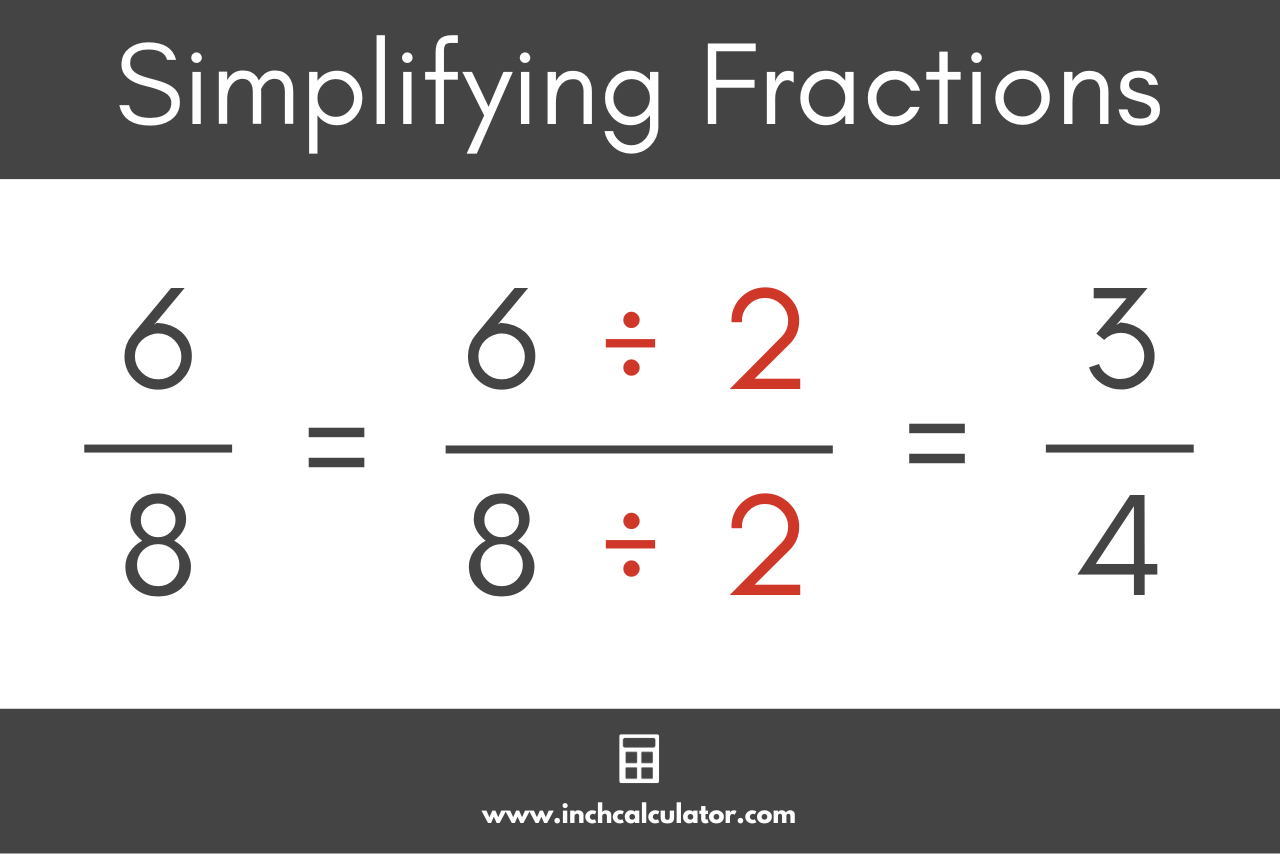### Step One: Find the Greatest Common Factor

The first step to reducing a fraction is to find the greatest common factor [GCF] between the numerator and the denominator. You’ll need the greatest common factor in the second step.

The greatest common factor is the largest number that is evenly divisible, or evenly goes into both the numerator and denominator with no decimals.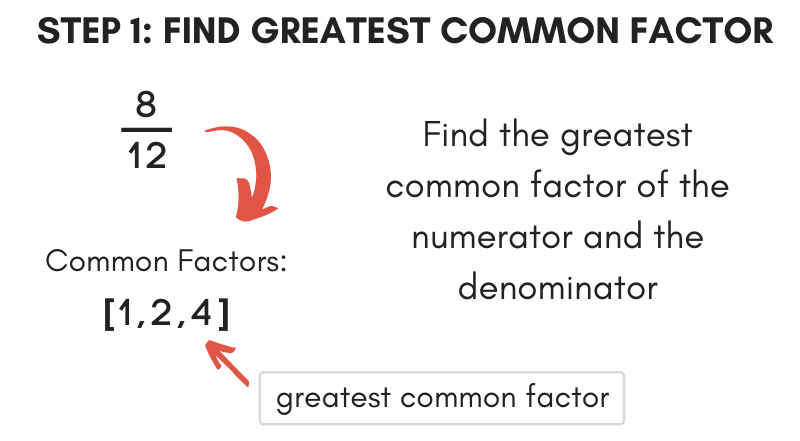For example, for the fraction is 6 / 8, let’s find the factors of 6 and 8.

The factors of 6 are [1,2,3,6].

The factors of 8 are [1,2,4,8].

The largest number common to both is 2; thus, 2 is the greatest common factor.

See our greatest common factor calculator for additional information on finding the greatest common factor.

### Step Two: Divide by the Greatest Common Factor

Armed with the greatest common factor, it’s now possible to reduce the fraction. To do so, divide both the numerator and the denominator by the greatest common factor. The result is a reduced fraction.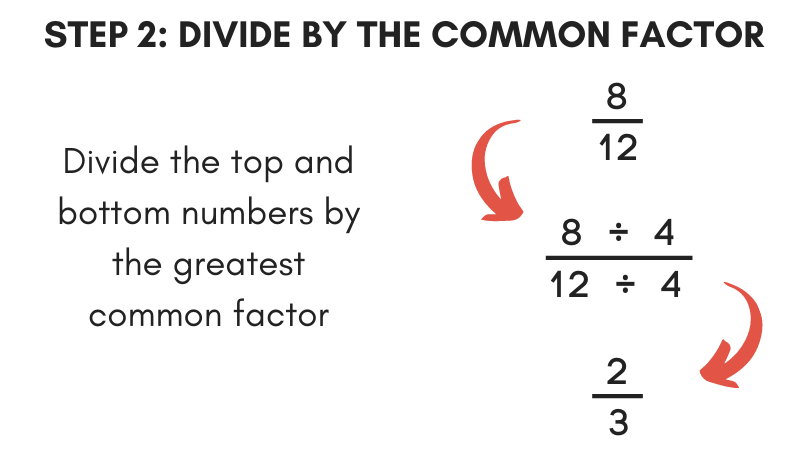Continuing the above example, simplify the fraction 6 / 8 by dividing the numerator and denominator by the greatest common factor of 2 found in the previous example.

6 / 8 = (6 ÷ 2) / (8 ÷ 2)
6 / 8 = 3 / 4

Thus, 3 / 4 is the simplified form of 6 / 8.

## The Easiest Way to Simplify Fractions

It’s fairly straightforward to reduce a fraction using the steps above, but there’s an even easier way to simplify fractions using a technique known as a division ladder.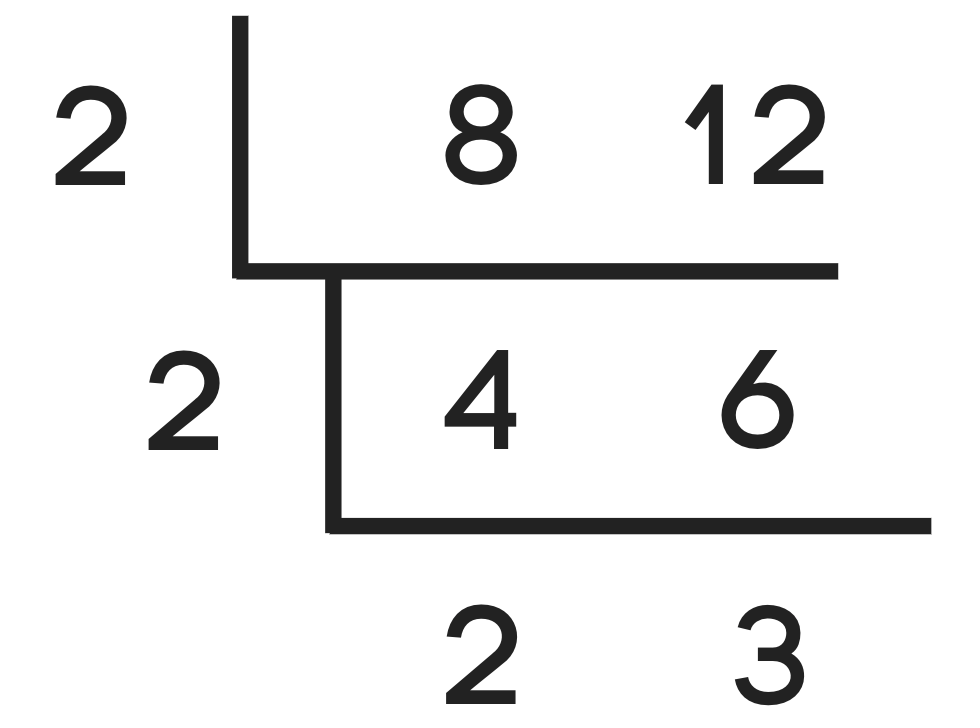To use a division ladder to simplify, start by placing the numerator and denominator next to each other, then draw an upside-down division line below and to the side of them.

Then, divide each number by the smallest common number between them. For instance, if both numbers are even, then divide them both by 2.

Continue doing this until there is no way to evenly divide both numbers by the same denominator. The numbers on the bottom represent the simplified fraction.

Let’s try using the division ladder to simplify 8/12 like the examples above.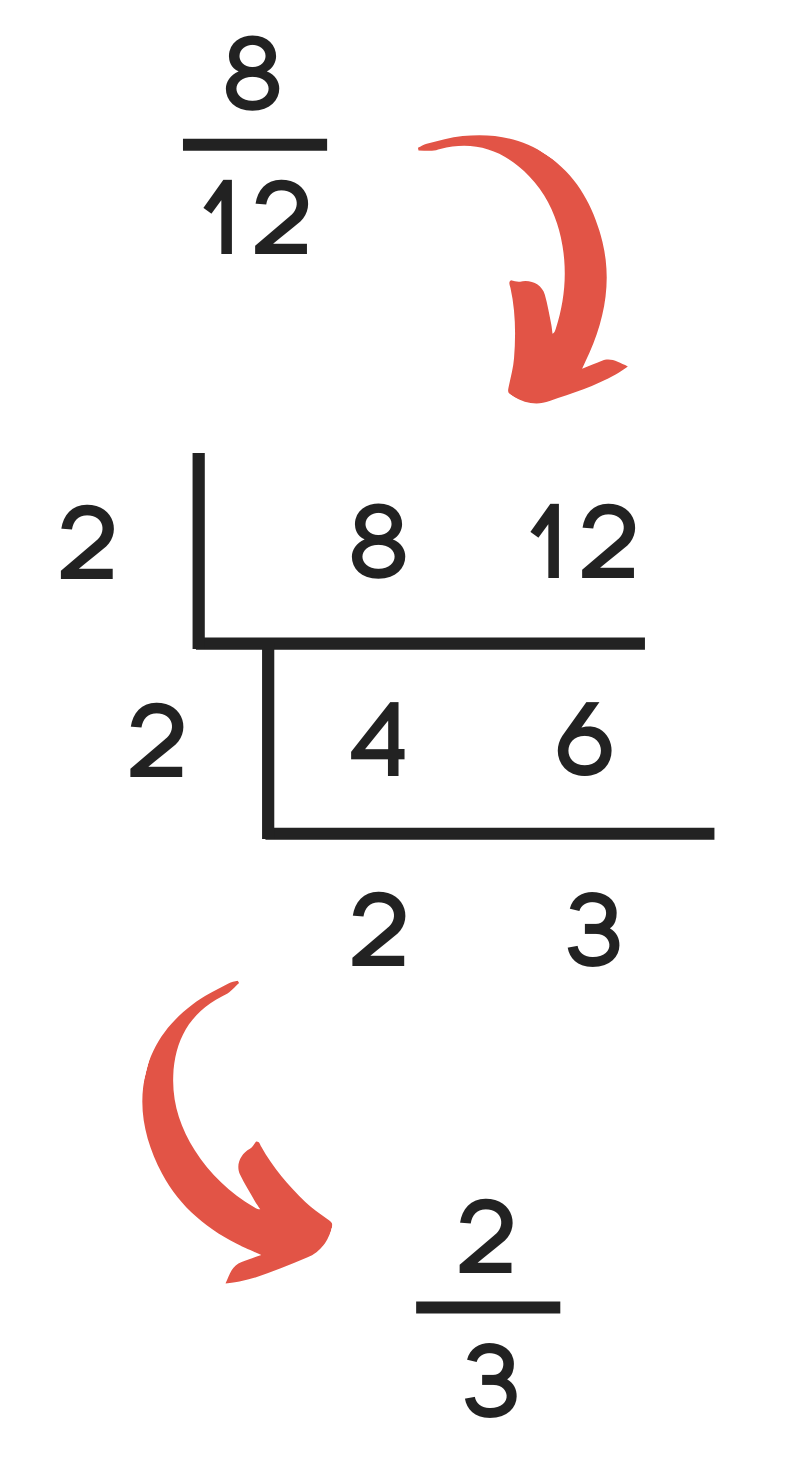As you can see, 8/12 can be reduced to 2/3 in just a few short steps using this method.

## How to Simplify and Reduce Improper Fractions

Some fractions are larger than 1, which is when the numerator is larger than the denominator. These are called improper fractions.

When the numerator is larger than the denominator, it is often helpful to simplify the improper fractions and then reduce the fraction further into a mixed number.

You can do this by dividing the numerator by the denominator, then using the quotient as the whole number and the remainder as the numerator of the new fraction with the original denominator.

For example, you can simplify 12 / 8 to 3 / 2. Now let’s convert this to a mixed number.

3 / 2 = 3 ÷ 2 = 1 R1
3 / 2 = 1 1 / 2

Here’s a tip: our fraction to mixed fraction calculator can help convert an improper fraction like this to a mixed number.

You can also use our fraction calculator to reduce a fraction.